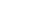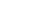# Physics Paper 3 Questions and Answers - Form 3 End Term 3 2022 Exams

## QUESTIONS

1.  You are provided with the following:
• A resistance wire mounted on mm scale.
• Two dry cells in a cell holder.
• A voltmeter.
• Four connecting wires, one with a crocodile clip at one end.

Proceed as follows:

1. Set –up the circuit as in the figure 1 below and determine the total electromotive force E of the cells.
Fig 1e.m.f of cells, E=_________________________________ volts . (1mark)
2. Set up the circuit shown in fig. 2 below, connect the wire with clip on the mounted wire at the length, L, of 10cm, from the end marked A. Record the voltmeter reading in the table provided in part C below.
Fig 23. Repeat the procedure in (b) above for the following values of length, L =20cm, 30cm, 40cm, 50cm, and 60cm. Complete the table 1 below. (7marks)
Table 1
 L (cm) Voltmeter reading V (V) E- V(V)102030405060
4. Plot a graph ofagainst L (cm). (5marks)5. Determine the slope of the graph. 2mks
6. Given that,=K1 L1 + Kdetermine the values of K1 and K2. (3 Marks)
7. Given that 4K2r =10, where r is the internal resistance of the cell. Determine the value of r. (2marks)

QUESTION 2
You are provided with the following:
Two retort stands each with a boss and clamp.

• A meter rule.
• A pendulum bob.
• A stop watch.
• A cotton thread 100cm long.
• Two pieces of wood.

Proceed as follows:

1. Use two pieces of wood on each clamp to hold the cotton thread on both of its ends as shown in figure 2(a).
2. Adjust the thread so that the distance x between points A and B is 100cm and then tighten the clamp to make a
firm grip.
3. Move the stands close to each other so that the distance x reduces to 90cm hence suspend the pendulum bob at the
center of the now sagging cotton thread as in fig.2(b).
4. Displace the bob sideways slightly and then release it so that it makes small oscillations in a direction perpendicular
to a vertical plane through AB.
5. Repeat the procedure b, c and d, for values of x indicated on the table and complete the table.Figure 2 (a) Figure 2 (b)

Table 2

 Distance x(cm) 90 80 70 60 50 40 Time for 10 oscillations t(s) PeriodT4 (S4) X2(cm2)

(10marks)
f) Use the grid provided to plot a graph of T4(vertical axis) against X2. (5marks)g)The relation between T and X is that T4= nx2+m where n and m are constants. Use your graph to determine:

1. n (3marks)
2. m (2marks)

## MARKING SCHEME

1.
1. E =3.0V± 0.2 (1mk)
2.
3.
 L(cm) V(volts) E-V(Volts) V        E-V 10 1.70 ± 0.2 1.3 1.3 20 1.90 ± 0.2 1.1 1.7 30 2.0 ± 0.2 1.0 1.0 40 2.1 ± 0.2 0.9 2.3 50 2.2 ± 0.2 0.8 2.8 60 2.3 ± 0.2 0.7 3.3 ½ x6=3mks 2mks all correct 2mks All correct
4.  S-1
A-1
P-2
L-1
5. Slope = 2.6 - 10
45-2.5
= 1.6
42.5
= 0.0376cm-1 (with units)
6. K1 = gradient for realising
= 0.0376 for candidate’s value
K2 = y- intercept
= 0.9 mark candidate’s value
7. 4x 0.9x r = 10
r = 10/4X0.9 =2.78 Ω for correct answer

2. (v)

 Distance x(cm) 90 80 70 60 50 40 Time for 10 oscillations, t (s) 9.85 16.36 12.29 13.00 13.22 13.75 ±234mks Period2mks T4(S4) 2mks X2 (cm)2 2mks

vi) Graph-5mks
A- 1mk
S -1mk
P - 2mks
L-1mk
vii) n-(3mks) correctly calculated to (3 dp)
m-(2mks) correctly calculated to (3 dp)

## CONFIDENTIAL

1. Each candidate require the following:
• A resistance wire mounted on a mm scale.(Nichrome wire SWG 32)
• Two dry cells in a cell holder.
• A voltmeter.(0-5v)
• Four connecting wires, one with a crocodile clip at one end.
2. Each candidate requires the following:
• Two retort stands each with a boss and clamp.
• A metre rule.
• A pendulum bob.
• A stop watch.
• A cotton thread (100cm long)
• Two pieces of wood.

• ✔ To read offline at any time.
• ✔ To Print at your convenience
• ✔ Share Easily with Friends / Students